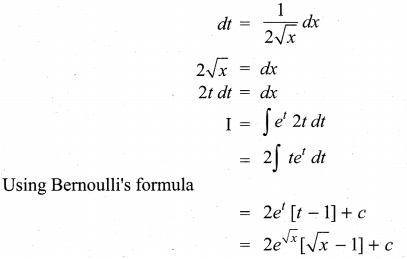# Samacheer Kalvi 11th Maths Solutions Chapter 11 Integral Calculus Ex 11.13

## Tamilnadu Samacheer Kalvi 11th Maths Solutions Chapter 11 Integral Calculus Ex 11.13

Choose the correct or most suitable answer from given four alternatives.
Question 1.
If $$\int f(x) d x$$ = g(x) + c, then $$\int f(x) g^{\prime}(x) d x$$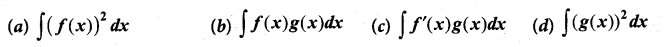Solution:
(a)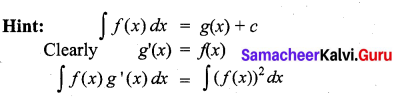Question 2.
If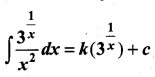, then the value of k is ……………
(a) log 3
(b) -log 3
(c) $$-\frac{1}{\log ^{3}}$$
(d) $$\frac{1}{\log 3}$$
Solution:
(c)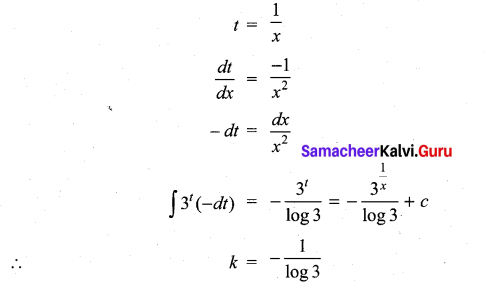Question 3.
If $$\int f^{\prime}(x) e^{x^{3}} d x$$ = (x – 1)ex2, then f(x) is …………………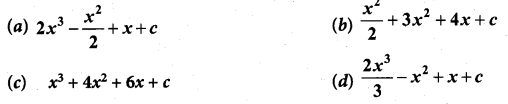Solution:
(d)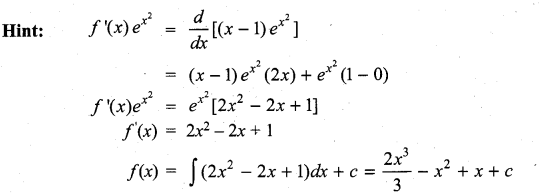Question 4.
The gradient (slope) of a curve at any point (x, y) is $$\frac{x^{2}-4}{x^{2}}$$. If the curve passes through the point(2, 7), then the equation of the curve is ………….
(a) y = x + $$\frac{4}{x}$$ + 3
(b) y = x + $$\frac{4}{x}$$ + 4
(c) y = x2 + 3x + 4
(d) y = x2 – 3x + 6
Solution: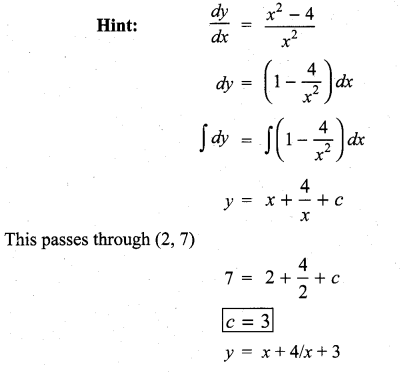Question 5.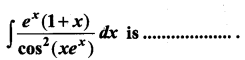(a) cot (xex) + c
(b) sec (xex) + c
(c) tan (xex) + c
(d) cos (xex) + c
Solution:
(c)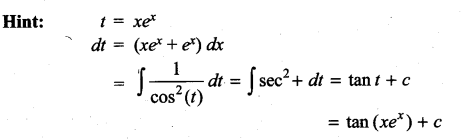Question 6.
$$\int \frac{\sqrt{\tan x}}{\sin 2 x} d x$$ is ……………..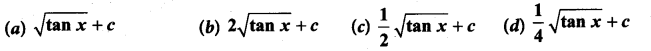Solution:
(a)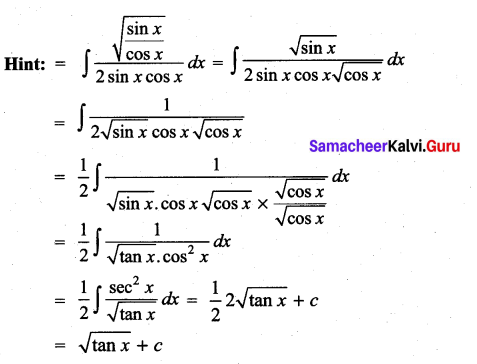Question 7.
$$\int \sin ^{3} x d x$$ is …………….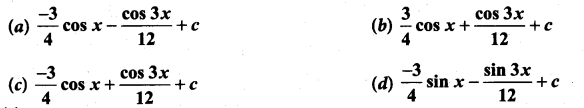Solution:
(c)
Hint: sin3x = $$\frac{1}{4}$$ (3 sin x – sin 3x)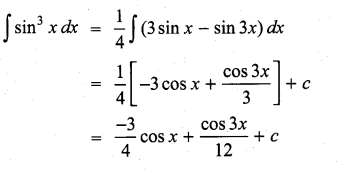Question 8.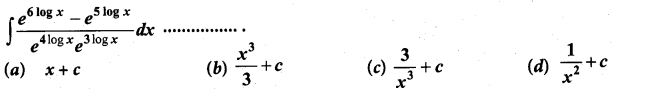Solution:
(b)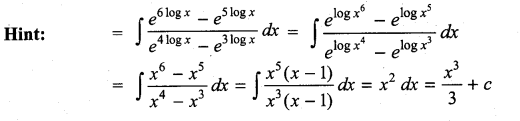Question 9.(a) tan-1 (sin x) + c
(b) 2 sin-1 (tan x) + c
(c) tan-1 (cos x) + c
(d) sin-1 (tan x) + c
Solution:
(d)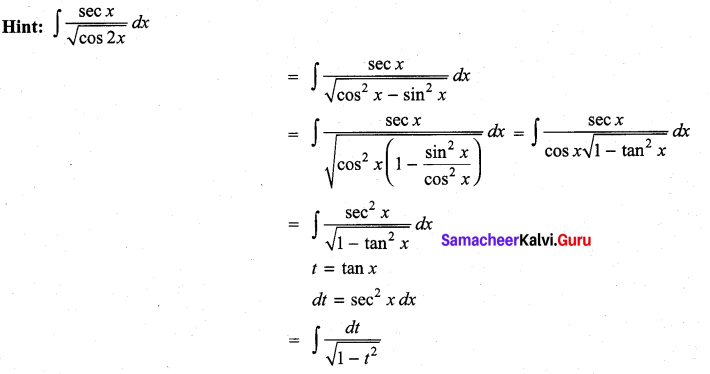= sin-1 (t) + c
= sin-1 (tan x) + cQuestion 10.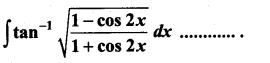(a) x2 + c
(b) 2x2 + c
(c) $$\frac{x^{2}}{2}$$ + c
(d) $$-\frac{x^{2}}{2}$$ + c
Solution:
(c)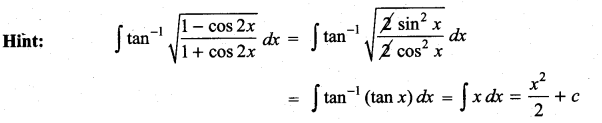Question 11.
$$\int 2^{3 x+5} d x$$ is ……………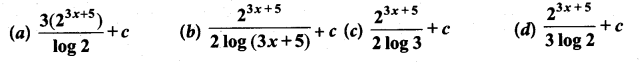Solution:
(d)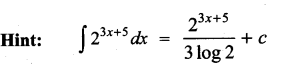Question 12.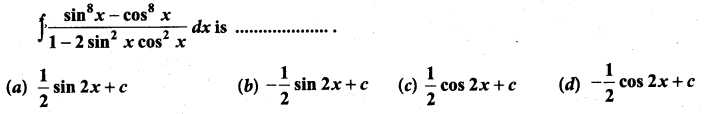Solution:
(b)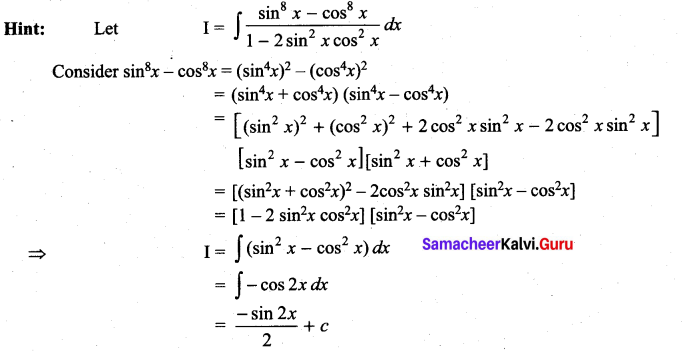Question 13.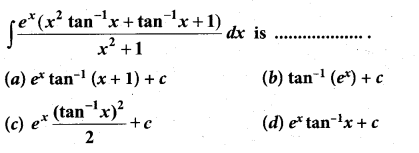Solution:
(d)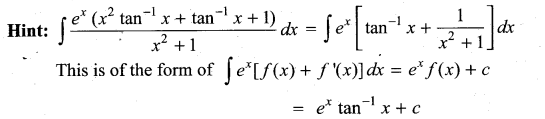Question 14.
$$\int \frac{x^{2}+\cos ^{2} x}{x^{2}+1}$$ cosec2xdx is …………….
(a) cot x + sin-1 x + c
(b) -cot x + tan-1 x + c
(c) -tan x + cot-1 x + c
(d) -cot x – tan-1 x + c
Solution:
(d)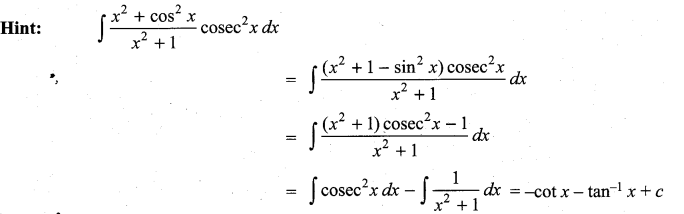Question 15.
$$\int x^{2} \cos x d x$$ is ……………
(a) x2 sin x + 2x cos x – 2 sin x + c
(b) x2 sin x – 2x cos x – 2 sin x + c
(c) -x2 sin x + 2x cos x + 2 sin x + c
(d) -x2 sin x – 2x cos x + 2 sin x + c
Solution:
(a)
Hint: $$\int x^{2} \cos x d x$$
By Bernoullis formula dv = cosxdx
u = x2 v = sinx
u’ = 2x v1 = -cos x
u” = 2 v2 = -sinx
= uv – u’v1 + u”v2
= x2sin x + 2x cos x – 2 sin x + cQuestion 16.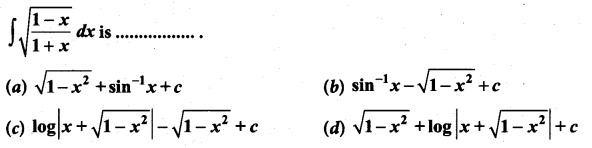Solution:
(b)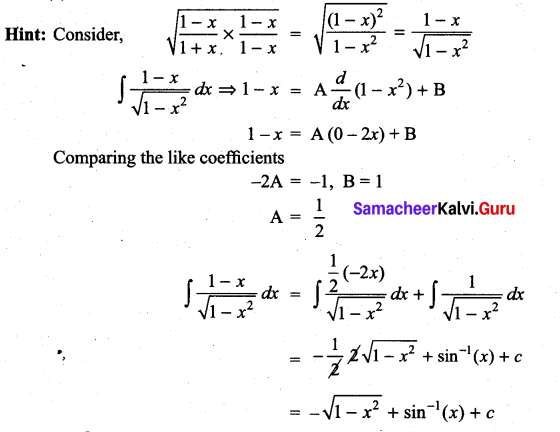Question 17.
$$\int \frac{d x}{e^{x}-1}$$ is …………….
(a) log |ex| – log |ex – 1| + c
(b) log |ex| + log |ex – 1| + c
(c) log |ex – 1| – log |ex| + c
(d) log |ex + 1| – log |ex| + c
Solution:
(c)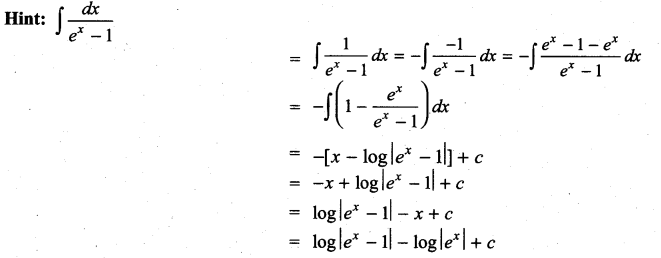Question 18.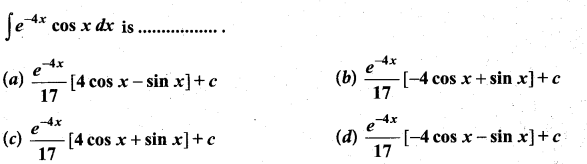Solution:
(b)
We know that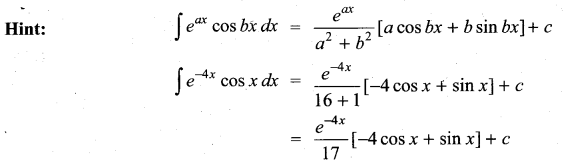Question 19.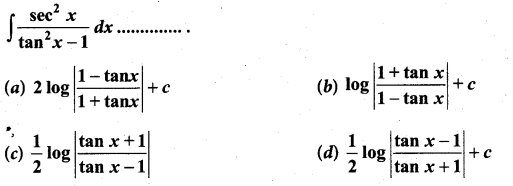Solution:
(d)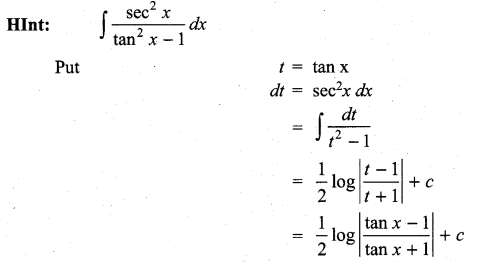Question 20.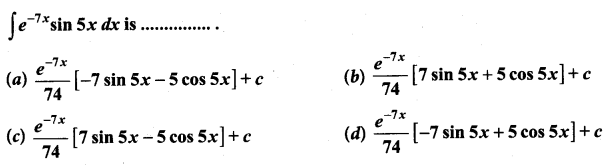Solution:
(a)
We know that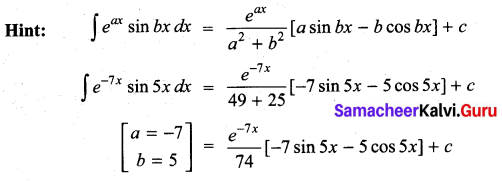Question 21.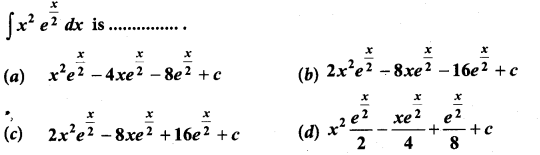Solution:
(c)
Hint: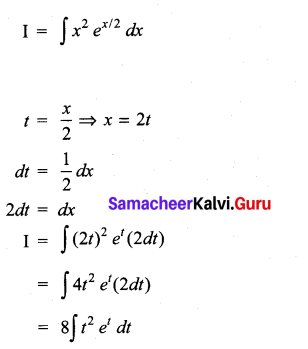By Bernoullis formula,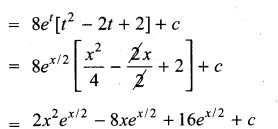Question 22.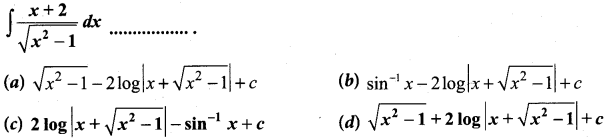Solution:
(d)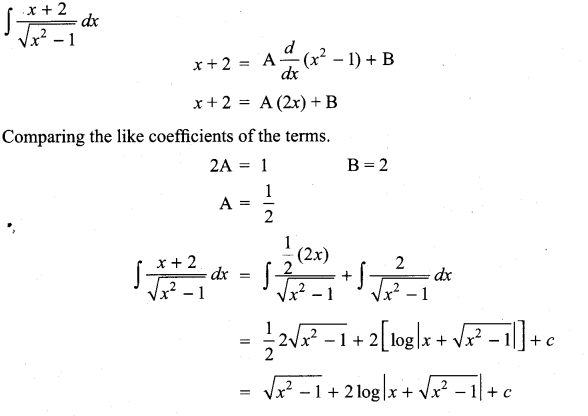Question 23.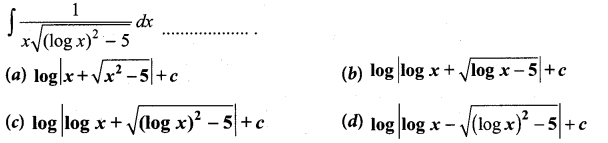Solution:
(c)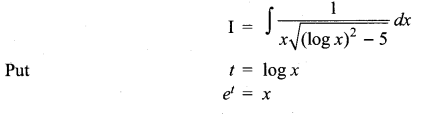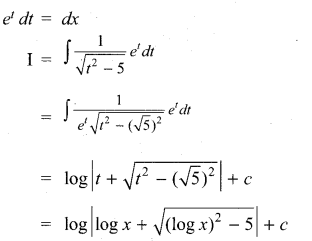Question 24.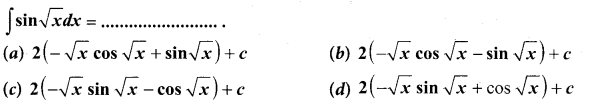Solution:
(a)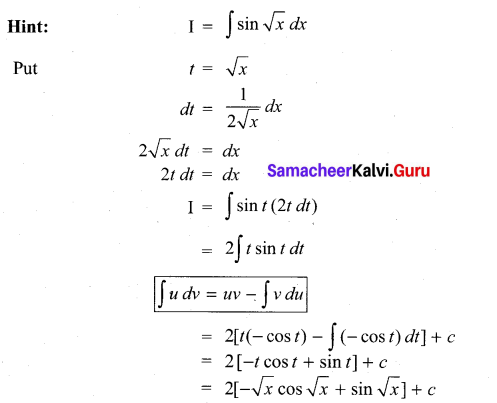Question 25.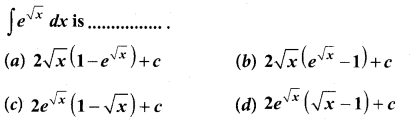Solution:
(d)
Hint: Let I = $$\int e^{\sqrt{x}} d x$$
t = $$\sqrt{x}$$